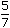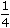# Time and Distance Questions Answers MCQ | Quantitative Aptitude for preparation of Competitive Exams

Time & Distance Questions Answers MCQ, Quantitative Aptitude, IBPS, SSC, Aptitude Test, IT Officer Test, Bank Exam, Objective, Interview, Time And Distance Problems With Solutions PDF, Time And Distance Solved Problems, Time And Distance Problems With Solutions Pdf For Bank Exams, Time And Distance Tricks, Time And Distance Questions For Bank PO, Time And Distance Concepts, Problems On Speed Distance And Time For Class 7, Time And Distance Formula, Practice Time, Speed & Distance Questions: Aptitude, Tricks To Solve The Time Speed Distance Questions, Time And Distance Problems With Solutions, How To Solve Time And Distance Questions, Speed Time And Distance Methods Shortcut Tricks

## Time and Distance Questions Answers MCQ | Quantitative Aptitude for preparation of Competitive Exams

1.

An aeroplane covers a certain distance at a speed of 240 kmph in 5 hours. To cover the same distance in 1hours, it must travel at a speed of:
 A. 300 kmph B. 360 kmph C. 600 kmph D. 720 kmph

2.

A train can travel 50% faster than a car. Both start from point A at the same time and reach point B 75 kms away from A at the same time. On the way, however, the train lost about 12.5 minutes while stopping at the stations. The speed of the car is:
 A. 100 kmph B. 110 kmph C. 120 kmph D. 130 kmph

3.

A man complete a journey in 10 hours. He travels first half of the journey at the rate of 21 km/hr and second half at the rate of 24 km/hr. Find the total journey in km.
 A. 220 km B. 224 km C. 230 km D. 234 km

4.

A car travelling withof its actual speed covers 42 km in 1 hr 40 min 48 sec. Find the actual speed of the car.
A.
 17 6 km/hr 7
B.25 km/hr
C.30 km/hr
D.35 km/hr

5.

It takes eight hours for a 600 km journey, if 120 km is done by train and the rest by car. It takes 20 minutes more, if 200 km is done by train and the rest by car. The ratio of the speed of the train to that of the cars is:
 A. 2 : 3 B. 3 : 2 C. 3 : 4 D. 4 : 3

Each of the questions given below consists of a statement and / or a question and two statements numbered I and II given below it. You have to decide whether the data provided in the statement(s) is / are sufficient to answer the given question. Read the both statements and

• Give answer (A) if the data in Statement I alone are sufficient to answer the question, while the data in Statement II alone are not sufficient to answer the question.
• Give answer (B) if the data in Statement II alone are sufficient to answer the question, while the data in Statement I alone are not sufficient to answer the question.
• Give answer (C) if the data either in Statement I or in Statement II alone are sufficient to answer the question.
• Give answer (D) if the data even in both Statements I and II together are not sufficient to answer the question.
• Give answer(E) if the data in both Statements I and II together are necessary to answer the question.

6.

 How much time did X take to reach the destination? I. The ratio between the speed of X and Y is 3 : 4. II. Y takes 36 minutes to reach the same destination.
 A. I alone sufficient while II alone not sufficient to answer B. II alone sufficient while I alone not sufficient to answer C. Either I or II alone sufficient to answer D. Both I and II are not sufficient to answer E. Both I and II are necessary to answer

7.

 The towns A, B and C are on a straight line. Town C is between A and B. The distance from A to B is 100 km. How far is A from C? I. The distance from A to B is 25% more than the distance from C to B. II. The distance from A to C isof the distance C to B.
 A. I alone sufficient while II alone not sufficient to answer B. II alone sufficient while I alone not sufficient to answer C. Either I or II alone sufficient to answer D. Both I and II are not sufficient to answer E. Both I and II are necessary to answer

8.

 Two towns are connected by railway. Can you find the distance between them? I. The speed of the mail train is 12 km/hr more than that of an express train. II. A mail train takes 40 minutes less than an express train to cover the distance.
 A. I alone sufficient while II alone not sufficient to answer B. II alone sufficient while I alone not sufficient to answer C. Either I or II alone sufficient to answer D. Both I and II are not sufficient to answer E. Both I and II are necessary to answer

9.

 Two cars pass each other in opposite direction. How long would they take to be 500 km apart? I. The sum of their speeds is 135 km/hr. II. The difference of their speed is 25 km/hr.
 A. I alone sufficient while II alone not sufficient to answer B. II alone sufficient while I alone not sufficient to answer C. Either I or II alone sufficient to answer D. Both I and II are not sufficient to answer E. Both I and II are necessary to answer

1.

Distance = (240 x 5) = 1200 km.

Speed = Distance/Time

Speed = 1200/(5/3) km/hr.     [We can write 1hours as 5/3 hours]Required speed =1200 x 3km/hr = 720 km/hr. 5

2.

Let speed of the car be x kmph.

 Then, speed of the train = 150 x =3 xkmph. 100 275 – 75 = 125 x (3/2)x 10 x 6075 – 50 = 5 x x 24x =25 x24= 120 kmph. 5

3.

 (1/2)x + (1/2)x = 10 21 24x + x = 20 21 2415x = 168 x 20x =168 x 20= 224 km. 15

4.

 Time taken = 1 hr 40 min 48 sec = 1 hr 40 4 min = 1 51 hrs = 126 hrs. 5 75 75

Let the actual speed be x km/hr.

 Then, 5 x x 126 = 42 7 75x =42 x 7 x 75= 35 km/hr. 5 x 126

5.

Let the speed of the train be x km/hr and that of the car be y km/hr.

 Then, 120 + 480 = 81 + 4 = 1 ….(i) x y x y 15
 And, 200 + 400 = 251 + 2 = 1 ….(ii) x y 3 x y 24

Solving (i) and (ii), we get: x = 60 and y = 80.Ratio of speeds = 60 : 80 = 3 : 4

6.

Since ratio of speed of X : Y is 3 : 4, then ratio of time will be 4 : 3.

I. If Y takes 3 min, then X takes 4 min.

 II. If Y takes 36 min, then X takes4 x 36min = 48 min. 3

Thus, I and II together give the answer.Correct answer is (E)

7.

I. AB = 125% of CB100 = 125 x (100 – x) 100100 – x = 100 x 100 = 80 125x = 20 km.AC = 20 km.

Thus, I alone gives the answer.

 II. AC = 1 CB 4x = 1 (100 – x) 45x = 100

x = 20.AC = 20 km.

Thus, II alone gives the answer.Correct answer is (C).

8.

Let the distance between the two stations be x km.

I. Then, speed of the mail train = (y + 12) km/hr.

 II. x – x = 40 . y (y + 12) 60

Thus, even I and II together do not give x.Correct answer is (D)

9.

I gives, relative speed = 135 km/hr.Time taken = 500 hrs. 135

II does not give the relative speed.I alone gives the answer and II is irrelevant.Correct answer is (A).